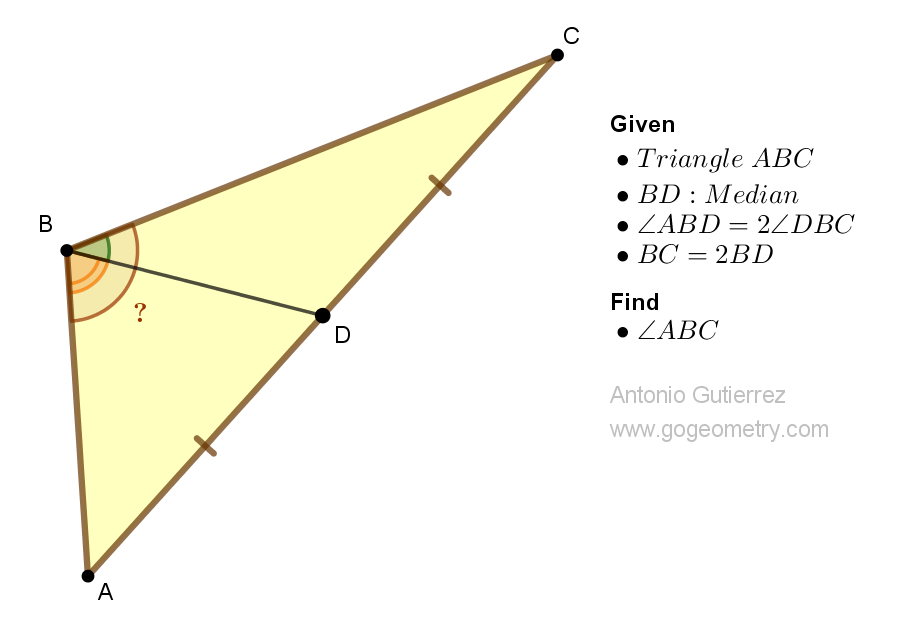## Tuesday, March 7, 2023

### Geometry Problem 1522: Unlocking the Angle Measure of a Triangle with Median and Doubled Side Lengths. Difficulty Level: High School.

Geometry Problem 1522. Post your solution in the comment box below.
Level: Mathematics Education, K-12 School, Honors Geometry, College.

Details: Click on the figure below.More Details

To post a solution to this problem click Comment underneath the post, or click into the line that says, “Enter Comment.” Type what you want to say and press Publish to post your solution.

1.Let E be the mid point of BC. Let <DBC = @ so that < ABD = 2@

Now BE = EC = BD
So Triangle BDE is isosceles and <BED = <BDE = 90 - @/2

From the midpoint theorem for Triangle ABC,
DE//AB so < ABD = 2@ = < BDE = 90 - @/2
Hence 5@/2 = 90 and so @ = 36

Therefore < ABD = 2@ = 72

Sumith Peiris
Moratuwa
Sri Lanka

1.< ABC = 3@ = 108